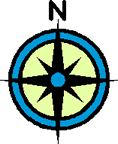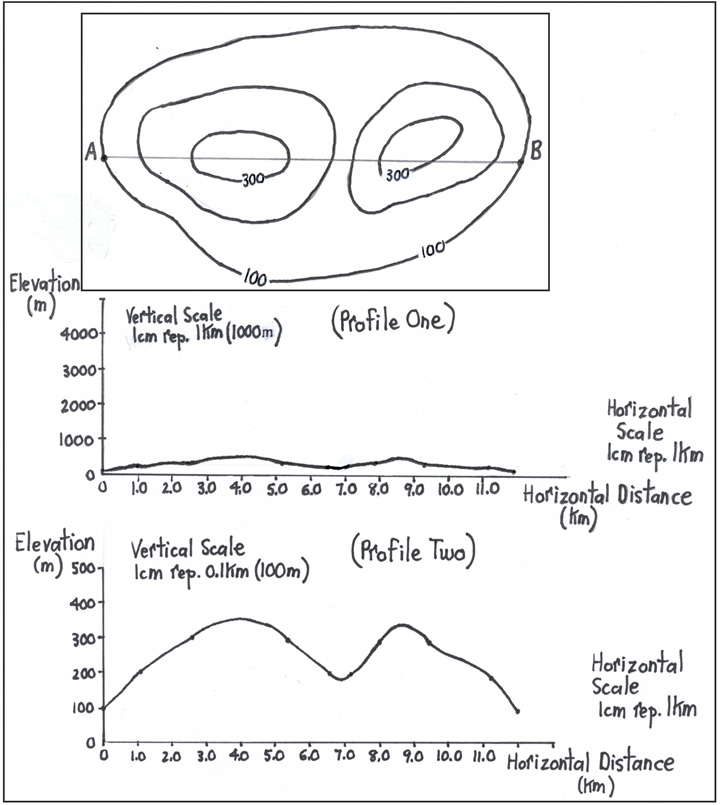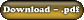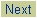Vertical Exaggeration
Objectives

To practice drawing cross-section profiles. To learn about the concept of vertical exaggeration and how to calculate it.Let's practice drawing cross-section profiles and learn what vertical exaggeration is.

 A true profile for A to B on the map below would look like Profile One.With a true profile, the vertical and horizontal scales are the same. However, as a result it is difficult to see the differences in slope. Hence, it is often useful to exaggerate the profile vertically by using a different vertical scale from the horizontal scale. For instance, using a vertical scale of 1cm represents 0.1km (100m) for the same map, yields Profile Two. Clearly, it is much easier to see the changes in slope along the profile. One can calculate how much this profile has been stretched vertically in comparison to the true profile. This is known as the Vertical Exaggeration. The formula for this is: Horizontal Scale Vertical Scale [Note: An easy way to remember that the Horizontal Scale goes on top is that "H" comes before "V" in the alphabet.] In this example, the Vertical Exaggeration would be: 1000m or 1 km 100m or 0.1 km = 10 times Therefore, Profile Two is stretched vertically 10 times its true profile (i.e. Profile One).

 Assignment Work 1) Download and complete the Vertical Exaggeration and Profiles Exercise.2) Check over your answers afterwards using the key.©www.coolschool.ca Press thebutton on the Action menu to proceed.